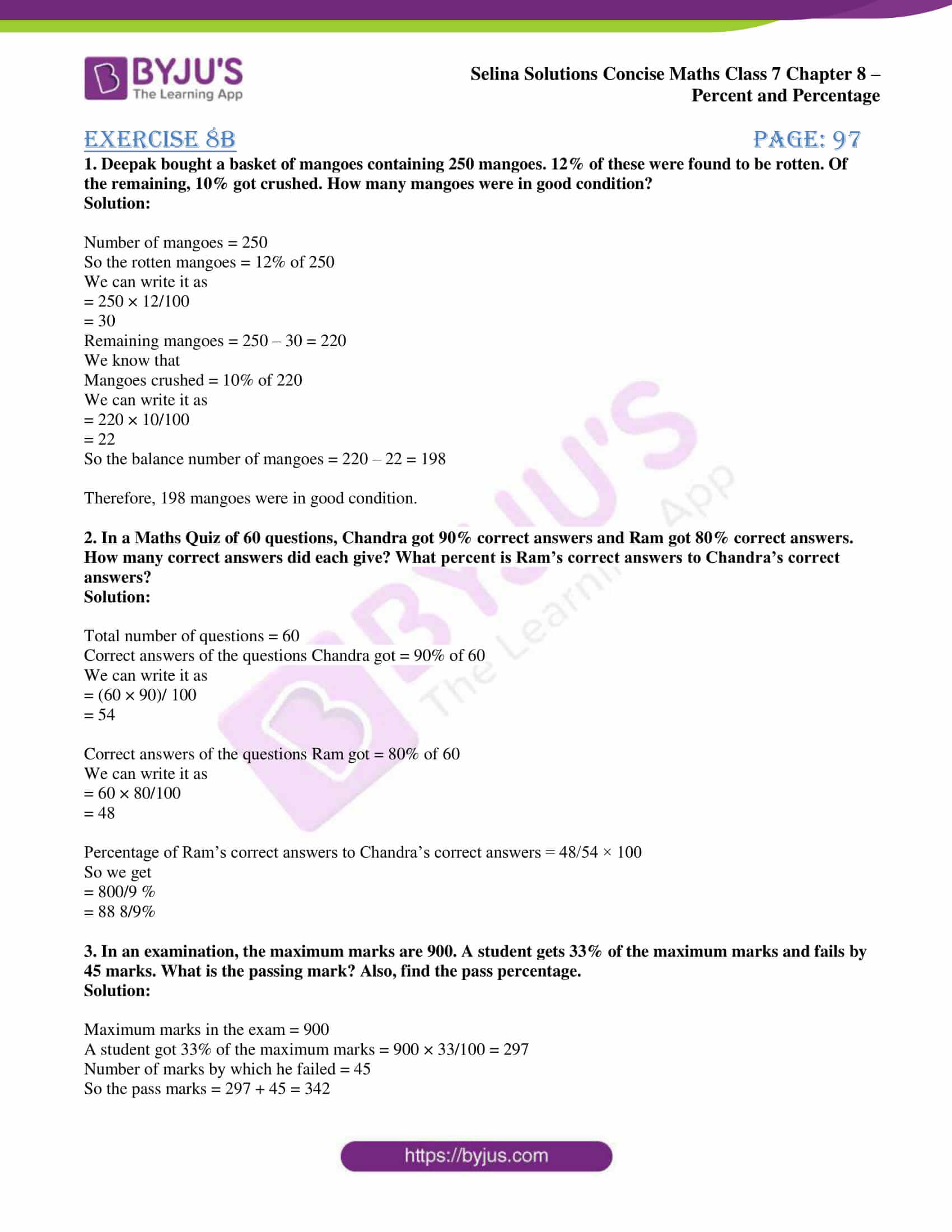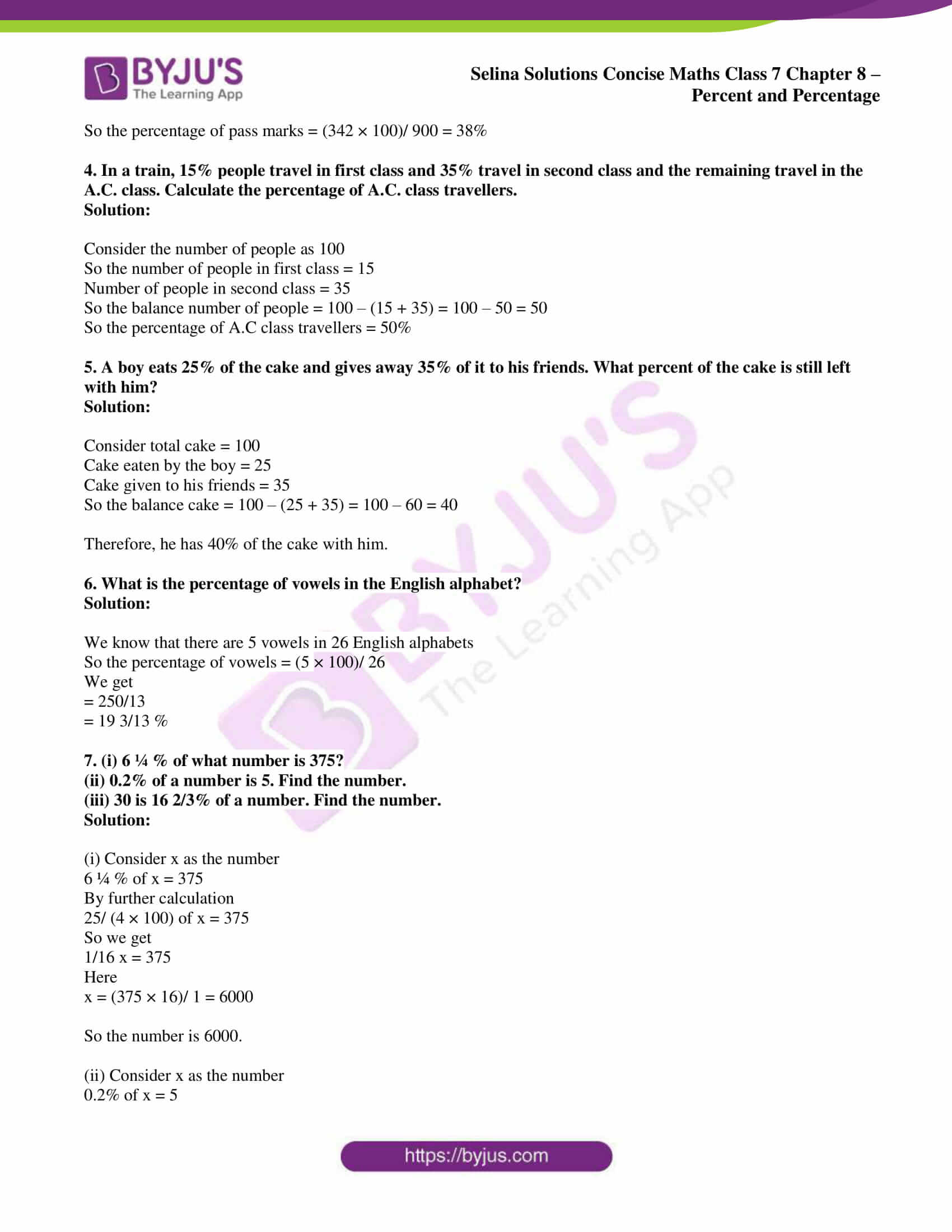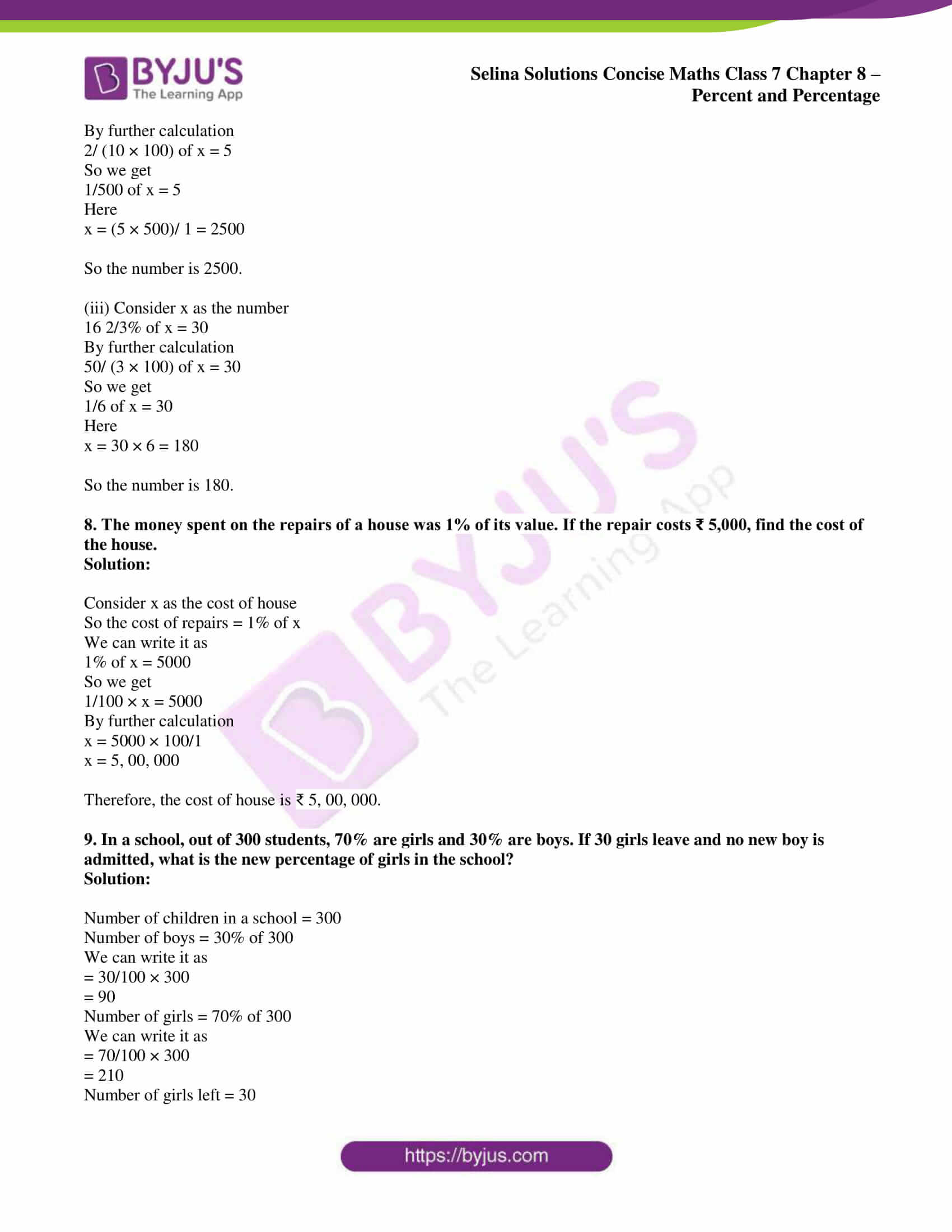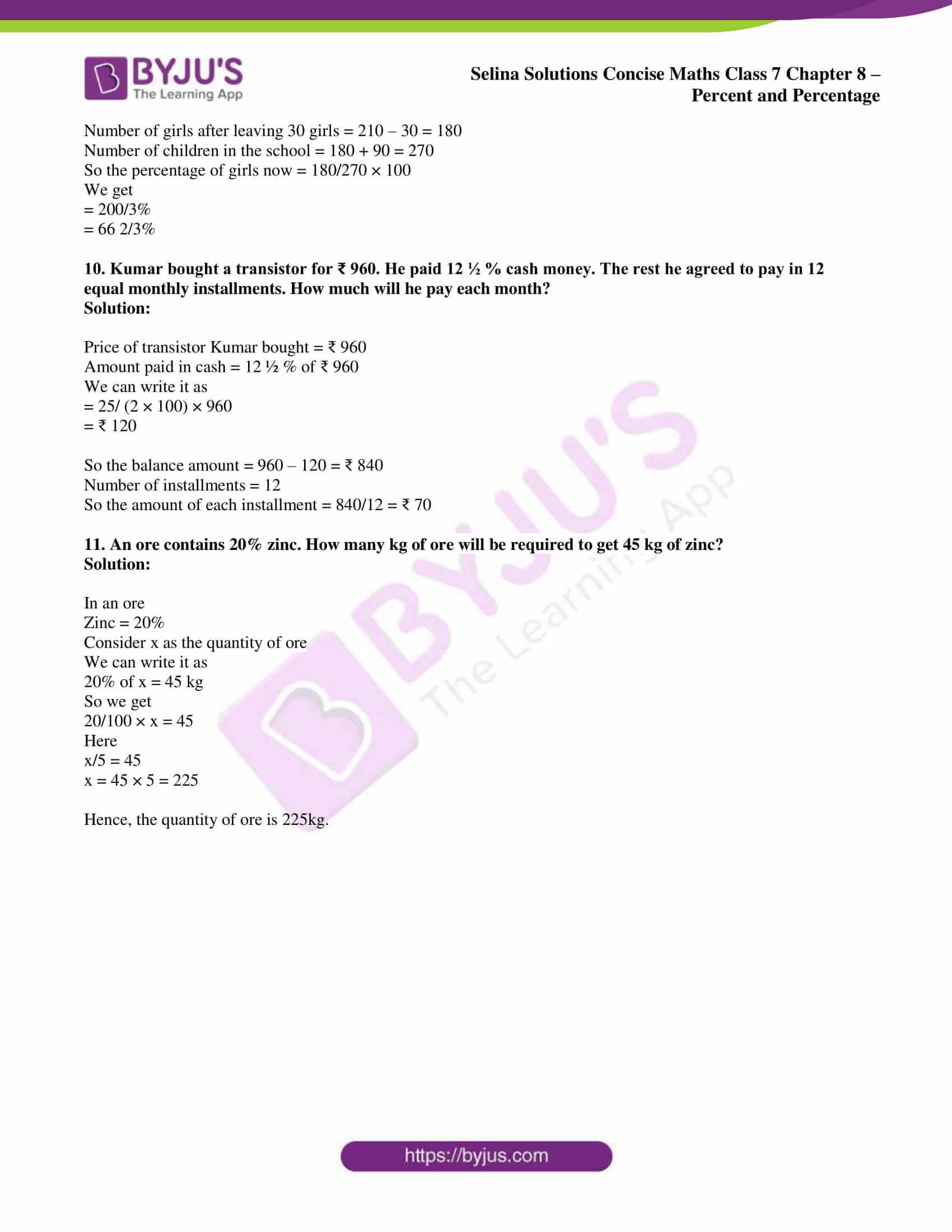# Selina Solutions Concise Maths Class 7 Chapter 8: Percent and Percentage Exercise 8B

Selina Solutions Concise Maths Class 7 Chapter 8 Percent and Percentage Exercise 8B has numerous solved examples in order to help students analyse the type of problems that would appear in the exam. The solutions contain explanations in simple language that propel students to perform well in the Class 7 annual exam. The detailed solutions assist students clear their confusions that arise while solving problems. Students can evaluate their performance by using Selina Solutions Concise Maths Class 7 Chapter 8 Percent and Percentage Exercise 8B free PDF, from the links available here.

## Selina Solutions Concise Maths Class 7 Chapter 8: Percent and Percentage Exercise 8B Download PDF### Access Selina Solutions Concise Maths Class 7 Chapter 8: Percent and Percentage Exercise 8B

#### Exercise 8B page: 97

1. Deepak bought a basket of mangoes containing 250 mangoes. 12% of these were found to be rotten. Of the remaining, 10% got crushed. How many mangoes were in good condition?

Solution:

Number of mangoes = 250

So the rotten mangoes = 12% of 250

We can write it as

= 250 × 12/100

= 30

Remaining mangoes = 250 – 30 = 220

We know that

Mangoes crushed = 10% of 220

We can write it as

= 220 × 10/100

= 22

So the balance number of mangoes = 220 – 22 = 198

Therefore, 198 mangoes were in good condition.

2. In a Maths Quiz of 60 questions, Chandra got 90% correct answers and Ram got 80% correct answers. How many correct answers did each give? What percent is Ram’s correct answers to Chandra’s correct answers?

Solution:

Total number of questions = 60

Correct answers of the questions Chandra got = 90% of 60

We can write it as

= (60 × 90)/ 100

= 54

Correct answers of the questions Ram got = 80% of 60

We can write it as

= 60 × 80/100

= 48

Percentage of Ram’s correct answers to Chandra’s correct answers = 48/54 × 100

So we get

= 800/9 %

= 88 8/9%

3. In an examination, the maximum marks are 900. A student gets 33% of the maximum marks and fails by 45 marks. What is the passing mark? Also, find the pass percentage.

Solution:

Maximum marks in the exam = 900

A student got 33% of the maximum marks = 900 × 33/100 = 297

Number of marks by which he failed = 45

So the pass marks = 297 + 45 = 342

So the percentage of pass marks = (342 × 100)/ 900 = 38%

4. In a train, 15% people travel in first class and 35% travel in second class and the remaining travel in the A.C. class. Calculate the percentage of A.C. class travellers.

Solution:

Consider the number of people as 100

So the number of people in first class = 15

Number of people in second class = 35

So the balance number of people = 100 – (15 + 35) = 100 – 50 = 50

So the percentage of A.C class travellers = 50%

5. A boy eats 25% of the cake and gives away 35% of it to his friends. What percent of the cake is still left with him?

Solution:

Consider total cake = 100

Cake eaten by the boy = 25

Cake given to his friends = 35

So the balance cake = 100 – (25 + 35) = 100 – 60 = 40

Therefore, he has 40% of the cake with him.

6. What is the percentage of vowels in the English alphabet?

Solution:

We know that there are 5 vowels in 26 English alphabets

So the percentage of vowels = (5 × 100)/ 26

We get

= 250/13

= 19 3/13 %

7. (i) 6 ¼ % of what number is 375?

(ii) 0.2% of a number is 5. Find the number.

(iii) 30 is 16 2/3% of a number. Find the number.

Solution:

(i) Consider x as the number

6 ¼ % of x = 375

By further calculation

25/ (4 × 100) of x = 375

So we get

1/16 x = 375

Here

x = (375 × 16)/ 1 = 6000

So the number is 6000.

(ii) Consider x as the number

0.2% of x = 5

By further calculation

2/ (10 × 100) of x = 5

So we get

1/500 of x = 5

Here

x = (5 × 500)/ 1 = 2500

So the number is 2500.

(iii) Consider x as the number

16 2/3% of x = 30

By further calculation

50/ (3 × 100) of x = 30

So we get

1/6 of x = 30

Here

x = 30 × 6 = 180

So the number is 180.

8. The money spent on the repairs of a house was 1% of its value. If the repair costs ₹ 5,000, find the cost of the house.

Solution:

Consider x as the cost of house

So the cost of repairs = 1% of x

We can write it as

1% of x = 5000

So we get

1/100 × x = 5000

By further calculation

x = 5000 × 100/1

x = 5, 00, 000

Therefore, the cost of house is ₹ 5, 00, 000.

9. In a school, out of 300 students, 70% are girls and 30% are boys. If 30 girls leave and no new boy is admitted, what is the new percentage of girls in the school?

Solution:

Number of children in a school = 300

Number of boys = 30% of 300

We can write it as

= 30/100 × 300

= 90

Number of girls = 70% of 300

We can write it as

= 70/100 × 300

= 210

Number of girls left = 30

Number of girls after leaving 30 girls = 210 – 30 = 180

Number of children in the school = 180 + 90 = 270

So the percentage of girls now = 180/270 × 100

We get

= 200/3%

= 66 2/3%

10. Kumar bought a transistor for ₹ 960. He paid 12 ½ % cash money. The rest he agreed to pay in 12 equal monthly installments. How much will he pay each month?

Solution:

Price of transistor Kumar bought = ₹ 960

Amount paid in cash = 12 ½ % of ₹ 960

We can write it as

= 25/ (2 × 100) × 960

= ₹ 120

So the balance amount = 960 – 120 = ₹ 840

Number of installments = 12

So the amount of each installment = 840/12 = ₹ 70

11. An ore contains 20% zinc. How many kg of ore will be required to get 45 kg of zinc?

Solution:

In an ore

Zinc = 20%

Consider x as the quantity of ore

We can write it as

20% of x = 45 kg

So we get

20/100 × x = 45

Here

x/5 = 45

x = 45 × 5 = 225

Hence, the quantity of ore is 225kg.